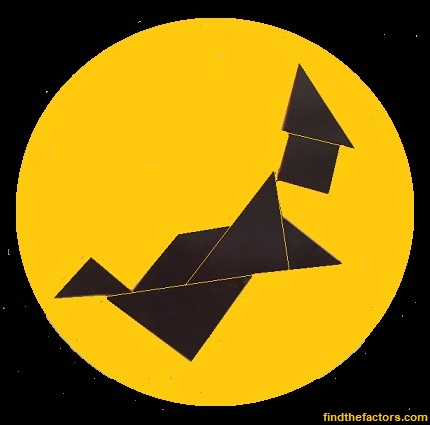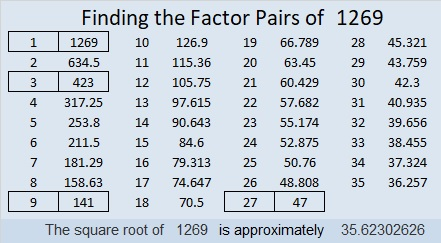# 1269 Tangram Witch Puzzle

If you have the seven tangram pieces, then you can create this Halloween witch riding across the moon (or a paper plate). Best Witches creating all kinds of things with those fabulous tiles!In case you would like to know some facts about the number 1269, here’s what I’ve learned:

• 1269 is a composite number.
• Prime factorization: 1269 = 3 × 3 × 3 × 47, which can be written 1269 = 3³ × 47
• The exponents in the prime factorization are 3 and 1. Adding one to each and multiplying we get (3 + 1)(1 + 1) = 4 × 2 = 8. Therefore 1269 has exactly 8 factors.
• Factors of 1269: 1, 3, 9, 27, 47, 141, 423, 1269
• Factor pairs: 1269 = 1 × 1269, 3 × 423, 9 × 141, or 27 × 47
• Taking the factor pair with the largest square number factor, we get √1269 = (√9)(√141) = 3√141 ≈ 35.623031269 is the difference of two squares four different ways:
37² – 10² = 1269
75² – 66² = 1269
213² – 210² = 1269
635² – 634² = 1269

This site uses Akismet to reduce spam. Learn how your comment data is processed.